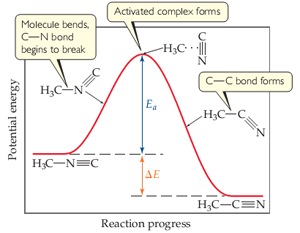# Problem: Suppose you could measure the rates for both the forward and reverse reactions of the process in the figure .In which direction would the rate be larger?

###### FREE Expert Solution

We have to determine whether the forward or reverse reaction would be faster using the given reaction energy diagram.

Activation energy is the minimum amount of energy required to initiate a chemical reaction.

The rate of a chemical reaction increases as the activation energy is lowered.

• Reactions will smaller activation energies are faster than reactions with larger activation energies.
88% (118 ratings)###### Problem Details

Suppose you could measure the rates for both the forward and reverse reactions of the process in the figure.

In which direction would the rate be larger?